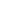Coarse Grained MD
Mechanical / Viscosity / Viscoelasticity
Materials Science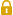User Only

We conducted deformation simulations for the stress-strain characteristics of rubber molecule models containing cross-linked structures to evaluate the effects of cross-linked structures and cross-linked atom types.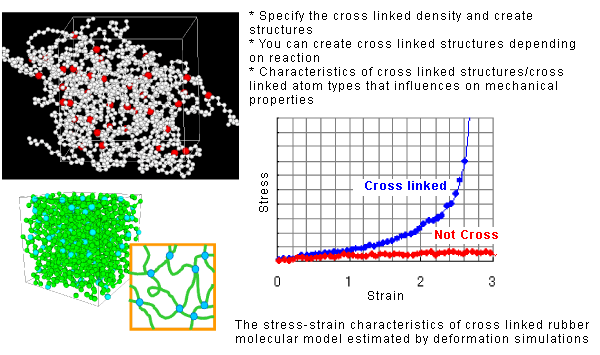Figure 1. Cross-Linked Rubber Deformation Simulation

Figure 2 shows a cross-linked structure created by the MD engine COGNAC. The cross-linked structure is created by inserting some cross-linker molecules into the existing bulk-state polymer and then calculating the reaction between the cross-linker and the monomer within the polymer.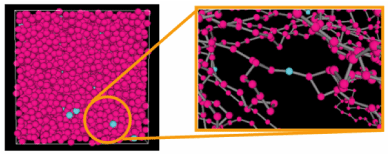Figure 2. Cross-Linked Polymer Structure.

Figure 3 shows an example of a uniaxial elongation calculation of a generated cross-linked structure at a constant speed. As the structure is stretched, the polymer chain changes its orientation to be more along the elongation direction.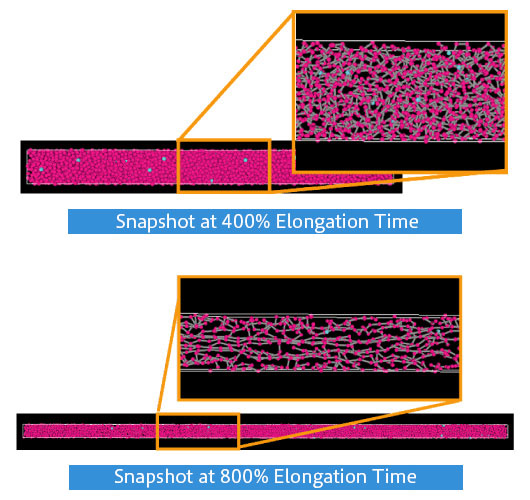Figure 3. Uniaxial Elongation Calculation of Cross-Linked Structure
Upper: Snapshot at 400% Elongation Time Lower: Snapshot at 800% Elongation Time

Figure 4 shows the stress-strain curve lines of a uniaxial elongation as the cross-link density change. Larger increases in the cross-link density or larger numbers of crosslinking points (N ν) results in polymer chains that are stretched at an earlier stage. This fact is reflected in the stress values.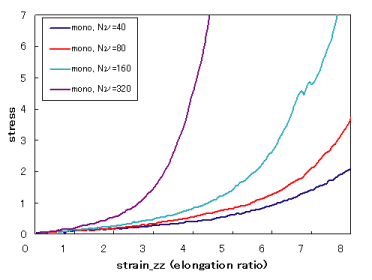Figure 4. Stress-Strain Characteristic and the Cross-Link Density Dependency